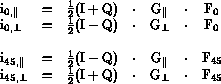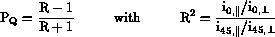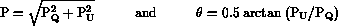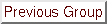### How to derive the Stokes parameters

The staring mode uses the calcite plate, which yields 2 spectra (of opposite polarization). The polarization information (one Stokes parameter per exposure) is contained in the ratio, at each wavelength, of the intensities in the 2 spectra but it is mixed up with the system gain ratio for the pixels concerned. The effect of the unknown gain is eliminated by inverting the sign of the polarization effects in a second exposure, while leaving the gain ratios identical. Inversion of (linear) polarization effects and therefore of the Stokes parameters is accomplished by rotating the halfwave plate by 45 degrees; while the polarization effects are inverted, the system gains remain the same since these are determined by the built-in polarization of the o and e exit beams of the calcite plate. All instrumental conditions (grating parameters, filters, dichroics, Dekker, slit etc) must be the same in both exposures; image centering on the slit is the most difficult to control in this respect.

The derivation of Stokes parameters from the recorded spectra is presented below. We factorise the conversion 'constant' for input flux to detector signal into a polarization-dependent, time-independent part G and a time-dependent, polarization-independent part F:

Gand Grefer to the o and e-spectra on a single frame; they include grating efficiencies and reflection coefficients of mirrors, and the sensitivity of the pixel considered to the polarized light striking it.
Fand Frefer to the two separate frames (halfwave at 0 and 45 degrees) and include atmospheric transmission, seeing, image wander and variations in shutter timing.
I and Q refer to total and polarized flux input and the i refer to signals recorded by the detector.
Pis the Q-component of the degree of polarization.

In this notation, we obtain:To derive Stokes parameters from these spectra, first divide the o and e ray spectra in each frame to take out the scaling factors F. Dividing these ratios again cancels the G factors. The Q Stokes parameter, in degree-of-polarization scale, is:Note that by multiplying the intermediate ratios, instead of dividing them, the G ratio (relative flat field) is obtained.

The other Stokes parameter,, is obtained similarly from the pair of exposures with the halfwave plate at 22.5 and 67.5 degrees. The raw degree of polarization P and polarization angle, are then given by:Note that this schematic procedure neglects CCD bias, sky background and calibration for instrumental parameters. Bias may be subtracted from the data frames as a first step or be treated as part of the sky background; sky subtraction and calibration are treated in separate subsections below.Previous: Spectropolarimetry
Up: Spectropolarimetry
Next: Imaging Polarimetry
Previous Page: Spectropolarimetry
Next Page: Imaging Polarimetry

Fri Jan 7 15:34:48 GMT 1994